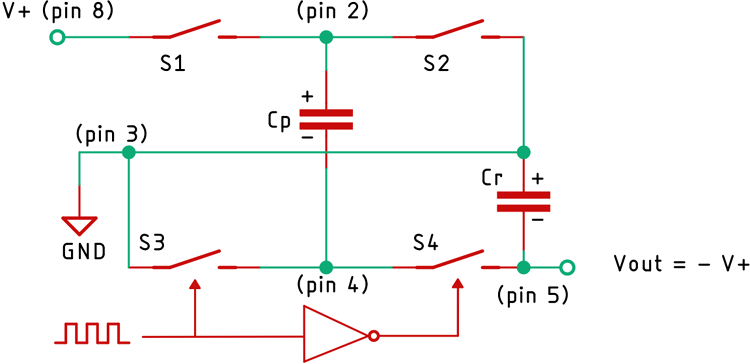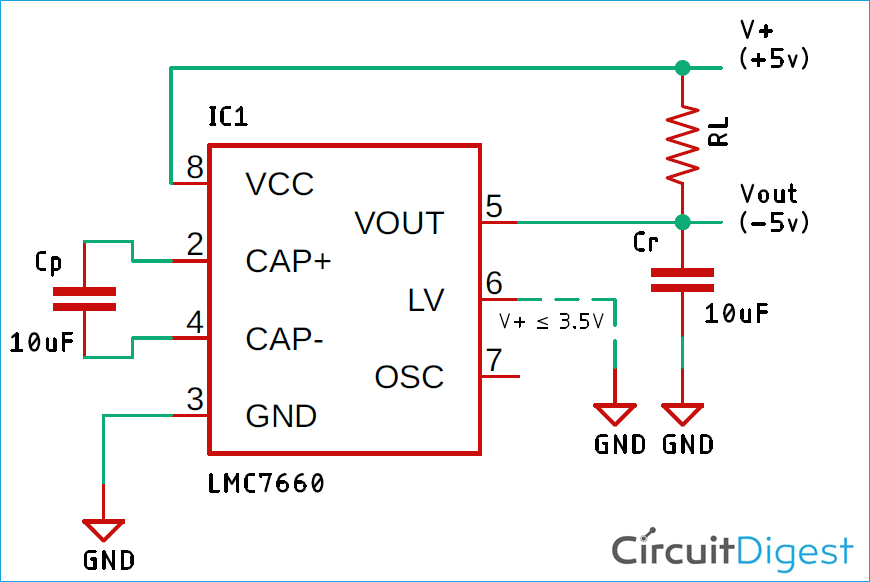# Switched Capacitor Voltage Converter

Published  February 25, 2020   0A switched capacitor voltage converter performs energy transfer and voltage conversion using capacitors. There are mainly two types of switched capacitor voltage converters, first is the voltage inverter circuit and the second is the voltage doubler circuit. These types of circuits are commonly known as charge pump circuits.

In this project, we will design a switched capacitor voltage inverter using Texas Instruments LMC7660S IC, and also build the circuit on a solderless breadboard to demonstrate its working. This circuit is very simple as it requires only two external capacitors to function properly.

### How does Switched Capacitor Voltage Inverter work?

Below is the functional block diagram of the 7600S IC, taken from its datasheet.The IC contains four large switches (mainly MOSFETs). In the first half of the input switching wave, switches S1 and S3 are closed, so it charges the pump capacitor Cp to supply voltage V+. During the second half of the switching wave, switches S2 and S4 are closed, and S1 and S3 are opened. As S2 connects the pump capacitor to ground, the output capacitor Cr develops a voltage which is -V+/2. After a few switching cycles, the voltage at the output capacitor will be exactly equal to -V+. At this point, the output voltage is the negative of the input voltage, and the input current is approximately equal to the output current.

Features:

Here is the feature list of the LMC7660 IC

• Simple Conversion of +10V Logic Supply to -10V Supplies
• Simple Voltage Multiplication (VOUT = (-) nVIN)
• Easy to Use - Requires Only 2 External Non-Critical Passive Components
• Typical Open Circuit Voltage Conversion Efficiency 99%
• Typical Power Efficiency 98%
• Wide Operating Voltage Range 1.5V to 10.0V
• No External Diode Over Full Temp. and Voltage Range

### Components Required

 Sl.No Parts Type Quantity 1 LMC7660 IC 1 2 10uF Capacitor 1 3 10K Resistor 1 4 Power Supply Unit DC (0 - 10)V 1 5 Single Gauge Wire Generic 6 6 Breadboard Generic 1

### Schematic Diagram

Below is the schematic for the Switched-Capacitor Voltage Converter taken from the datasheet.### Applications of Switched Capacitor Voltage Regulator IC LMC7660S

This is a very small, efficient and useful IC which can be used in various applications

• Suppose, you need to measure True RMS voltage with the help of a microcontroller. For that, you need an Op-Amp to amplify the input AC signal and a Dual polarity supply to power the Op-Amp. In this scenario, the LMC7660 IC can be very useful. By putting this IC and two inexpensive capacitors in your circuit, you can easily generate the Negative of the input voltage.
• In another scenario where you need to amplify a signal from a capacitive microphone, it also requires a dual polarity supply to amplify the signal properly. In this situation, the 7660 IC can come in very handy.
• To power, a Push-Pull amplifier dual polarity supply is required.
• On-Board Negative Supply for Dynamic RAMs.
• This IC is also used in the telecom industry where it is used to power an OP07 Op-Amp and an Analog Multiplexer CD4051, which is a very crucial part of the whole circuit as it is used to measure the battery voltage, Input AC voltage, and Input AC Current.

### Do’s And Don’ts

• The maximum input voltage of this IC is 10V, an input voltage higher than 10V will surely damage this IC.
• The Pin-6 of the IC is the LV (Low Voltage) Pin if the input voltage is ≤3.5V, this pin should be grounded. Otherwise, this pin should be in a floating state.
• The capacitor CP should be placed very close to the IC otherwise, the IC can latch-up.
• To increase the efficiency of the circuit a capacitor with low ESR ratings can be used.
• One thing to keep in mind is that efficiency decreases with an increase in load current, for example with a load current of 40 mA, the efficiency is around 75%.

I hope you liked this article and learned something new out of it. If you have any doubt, you can ask in the comments below or can use our forums for detailed discussion.

Video